# GSEB Solutions Class 11 Statistics Chapter 6 Permutations, Combinations and Binomial Expansion Ex 6

Gujarat Board Statistics Class 11 GSEB Solutions Chapter 6 Permutations, Combinations and Binomial Expansion Ex 6 Textbook Exercise Questions and Answers.

## Gujarat Board Textbook Solutions Class 11 Statistics Chapter 6 Permutations, Combinations and Binomial Expansion Ex 6

Section – A

Choose the correct option from those given below each question:

Question 1.
In one group there are m distinct things and in the other group there are n distinct things. In how many ways can one thing be selected from both the groups?
(a) mn
(b) $$\frac{m}{n}$$
(c) m – n
(d) m + n
(d) m + n

Question 2.
If the first action can be done in m ways and second action can be done in n ways then in how many ways can both the actions be done together?
(a) mn
(b) $$\frac{m}{n}$$
(c) m – n
(d) m + n
(a) mn

Question 3.
What is n !?
(a) Addition of 1 to n natural numbers
(b) Multiplication of 1 to n natural numbers
(c) Multiplication of 1 to n-r natural numbers
(d) Multiplication of 0 to n natural numbers
(b) Multiplication of 1 to n natural numbers

Question 4.
In usual notations, which of the following relation between permutation and combination is true?
(a) nCr = nPr × r !
(b) nPr = nCr + r !
(c) nPr = $$\frac{{ }^{n} C_{r}}{r !}$$
(d) nCr = $$\frac{n P_{r}}{r !}$$
(d) nCr = $$\frac{n P_{r}}{r !}$$

Question 5.
Which of the following is equivalent to nCr?
(a) $$\frac{n !}{(n-r) !}$$
(b) nCn-r
(c) nCr-1
(d) $$\frac{n_{r+1}}{r}$$
(b) nCn-r

Question 6.
Find the value of nC0 + nCn.
(a) 0
(b) 1
(c) 2
(d) 2n
(c) 2

Question 7.
If (n + 1) ! = 120 then find the value of n.
(a) 3
(b) 4
(c) 5
(d) 6
(b) 4Question 8.
How many terms are there in the expansion of (x + a)n-1?
(a) n
(b) n-2
(c) n + 1
(d) n + 2 .
(a) n

Question 9.
If 10 × n! = 240 then find the value of n.
(a) 6
(b) 3
(c) 5
(d) 4
(d) 4

Question 10.
State the last term in the expansion of (x + a)n.
(a) an
(b) an-1
(c) x0
(d) xn-1
(a) an

Question 11.
A ride in a fun fair has 8 seats. In how many ways can 3 persons be arranged in this ride?
(a) 8C3
(b) 3P8
(c) 3C8
(d) 8P3
(d) 8P3

Section – B

Answer the following questions in one sentence:

Question 1.
What is the main difference between permutation and combination?
Permutation means an arrangement of things in which order of arrangement is important, while combination means selection of things in which order of selection is not important.

Question 2.
Write the fundamental principle of counting for addition.
If there are m distinct things in Group 1 and n distinct things in Group 2, then selection of one thing from total things of both groups can be done in m + n ways. This rule is called the fundamental principle of counting for addition.

Question 3.
Write the fundamental principle of counting for multiplication.
If the first operation can be done in m ways and second operation can be done in n ways, then two operations together can be done in m x n ways. This rule is called the fundamental principle of counting for multiplication.

Question 4.
Write the mathematical relationship between permutation and combination in usual notations.
In usual notations the mathematical relationship between permutation and combination is : nPr = nCr . r !

Question 5.
Write the coefficients of the terms in the expansion of (x + a)n for n = 6.
The coefficients of the terms in the expansion of (x + a)n for n = 6 are 1, 6, 15, 20, 15, 6, 1.Question 6.
Write the general term of the expansion (x + a)n.
The general term of the expansion of (x + a)n is Tr+x = nCrxn-r an.

Question 7.
There are 5 empty seats in the coach of a train. In how many ways will 3 persons be seated?
In 5 empty seats in the coach of a train 3 persons can be seated in 5P3 = 5 × 4 × 3 = 60 ways.

Question 8.
If nC2 = 15, then find the value of n.
nC2 = 15
∴ $$\frac{n !}{2 !(n-2) !}$$ = 15
∴ $$\frac{n(n-1)(n-2) !}{2 \cdot(n-2) !}$$ = 15
∴ n(n – 1) = 15 × 2
∴ n(n- 1) = 30 = 6 × 5
∴ n[n – 1) = 6(6-1)
∴ n = 6

Question 9.
If nP3 = 210, find the value of n.
nP3 = 210
∴ $$\frac{n !}{(n-3) !}$$ = 210
∴ $$\frac{n(n-1)(n-2)(n-3) !}{(n-3) !}$$ = 210
∴ (n – 1)(n – 2)(n – 3) = 210
∴ (n – 1)(n – 2)(n – 3) = 7 × 6 × 5
= 7(7 – 1)(7 – 2)
∴ n = 7. Hence, n = 7

Question 10.
How many new arrangements can be made using all the letters of the word TUESDAY?
Using 7 letters of the word, total number of arrangement TUESDAY
= 7P7
= 7! = 7 × 6 × 5 × 4 × 3 × 2 × 1
= 5040

∴ New arrangements
= 5040 – 1 (Given arrangement TUESDAY)
= 5039

Question 11.
How many arrangements can be made using all the letters of the world VIAAN?
In 5 letters of the word VIAAN. A is repeated 2 times.
Number of arrangements = $$\frac{5 !}{2 !}=\frac{120}{2}$$ = 60.

Question 12.
In how many ways can 5 different letters be placed in 5 covers?
5 different letters can be place in 5 covers in 5P5 = 5! = 120 ways.Question 13.
What is nPr?
If r distinct things out of n distinct things are to be arranged in r different places, then total number of such arrangements is called nPr.

Question 14.
Write the coefficient of (n + 1) terms in the expansion of (x + a)n.
The coefficients of (n + 1) terms in the expansion of (x + a)n are : nC0, nC1, nC2, nC3, nC4,……..nCn-2, nCn-1.nCn

Question 15.
If nCx = nCy, then write the two possible relationships between x and y.
If nCx = nCy, then possible relationships between x and y are : (1) x = y (2) x + y = n.

Section – C

Answer the following questions as required :

Question 1.
Write the characteristics of binomial expansion.
Characteristics of binomial expansion :
The following characteristics are seen in the expansion of (x + a)n:

• Total number of terms in the expansion is (n + 1).
• The coefficients of these terms are nC0, nC2nCn respectively.
• The sum of the coefficient of these terms is 2n, i.e., nC0 + nC1 + nC2 + … + nCn = 2n.
• The first term is nC0xn = xn and last term is nCnan = an.
• In the successive terms, the power of ‘x’ goes on decreasing by one, while power of ‘a’ goes on increasing by one.
• In any term the sum of the powers of x and a is n.
• The coefficients of the terms equidistant from the middle term are equal.

Question 2.
10 schools participate in a science fair. In how many ways can the first, second and the third prizes be distributed among these schools?
Among 10 schools the first, second and third prizes can be distributed in 10P3 = 10 × 9 × 8 = 720 ways.

Question 3.
In how many ways can 4 boys and 3 girls be arranged In a row such that no two boys and no two girls are together?
4 boys and 3 girls can be arranged in a row in the order B G B G B G B so that no two boys and no two girls are together
∴ Total permutations = 4P4 × 3P3
= 4! × 3!
= 24 × 6
= 144

Question 4.
There are 6 different books of Statistics, 5 different books of Accounts and 4 different books of English on a table. In row many ways can these books be arranged in a row such that the books of same subject remain together?
The books of the subjects statistics, Accounts and English can be arranged in 3P3 ways.
6 different books of Statistics can be arranged in 6P6 ways.
5 different books of Accounts can be arranged in 5P5 ways and
4 different books of English can be arranged in 4P4 ways.

∴ Total permutations = 3P3 × (6P6 × 5P5 × 4P4)
= 3! × (6! × 5! × 4!)
= 6 × (720 × 120 × 24)
= 6 × 2073600
= 12441600

Question 5.
How many 5 dIgit numbers can be formed using all the dIgits 3, 8, 0, 7, 6?
To form 5 dIgit numbers using all the digits 3. 8. 0. 7, 6.
0 should not be In the first place.
∴ From the remaIning 4 dIgits (3. 8. 7.6) one digit can be placed at the first placed In ways.
Now, remaining 4 digIts including O, can be arranged In remaIning 4 places in 4P4 ways.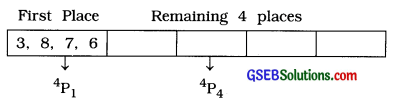Total permutations = 4P1 × 4P4
= 4 × 4!
= 4 × 24 = 96

Question 6.
In how many ways can all the letters of the word TANI be arranged so that vowels remain together?
There are 4 letters in the word TANI of which 2 vowels are A and I.Considering 2 vowels as 1 letter, total (2 + 1) = 3 letters can be arranged in 3P3 ways and in each of these arrangements two vowels can be arranged among themselves in 2P2 ways.

Total permutations = 3P3 × 2P2
= 3! × 2!
= 6 × 2 = 12

Question 7.
In how many ways can all the letters of the word MANGO be arranged so that vowels are not together?
There are 5 letters of the word MANGO of which two vowels are A and O.
Now, 5 letters of the word MANGO can be arranged in 5P5 = 5! = 120 ways.

Considering two vowels as one letter, total (3 + 1) 4 letters can be arranged in 4P4 ways and in each of these arrangements two vowels can be arranged in 2P2 ways.

∴ Toted permutations in which vowels are together
= 4P4 × 2P2
= 4! × 2!
= 24 × 2 = 48

Now, Total permutations in which two vowels are not together
= [Total permutations for arranging all 5 letters] – [Total permutations in which two vowels are together]
= [120 – 48] = 72Question 8.
How many numbers can be formed using all the digits of the number 1234321 such that odd digits occupy odd places only?
In the number 1234321 the odd digits are 1, 3, 3, 1 and even digits are 2, 4, 2.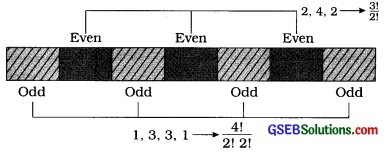4 odd digits can occupy odd places in $$\frac{41}{2 ! 2 !}$$ ways and 3 even digits can occupy the remaining even places in $$\frac{3 !}{2 !}$$ ways.

∴ Total permutations = $$\frac{4 !}{2 ! 2 !} \times \frac{3 !}{2 !}$$
= $$\frac{24}{2 \times 2} \times \frac{6}{2}$$
= 6 × 3
= 18

Question 9.
What will be the ratio of number of arrangements obtained using all the letters of the word ROLLS and DOLLS?
In 5 letters of the word ROLLS, the letter L comes two times.
Total permutations = $$\frac{5 !}{2 !}=\frac{120}{2}$$ = 60
In 5 letters of the word DOLLS, the letter L comes two times

Total permutations = $$\frac{5 !}{2 !}=\frac{120}{2}$$ = 60
∴ The ratio of number of arrangements of all the letters of the word ROLLS and DOLLS = $$\frac{60}{60}=\frac{1}{1}$$ = 1 : 1

Question 10.
There are 2 defective screws in a box having 6 screws. In how many ways can 2 non-defective screws be selected from the box?
2 screws are defective In a box of 6 screws.
∴ Non-defective screws In a box = 6 – 2 = 4
2 non-defective screws out of 4 non-defective screws can be selected in 4C2 = $$\frac{4 \times 3}{2 \times 1}$$ = 6 ways.

Question 11.
In how many ways can 2 cards of queen or king can be selected from a well shuffled pack of 52 cards?
In a pack of 52 cards, there are 4 cards of king and 4 cards of queen.
From well shuffled pack of 52 cards, 2 cards of queen
OR
2 cards of king can be selected in 4C2 +4C2 ways.
∴ Total combinations = 4C2 + 4C2
$$\frac{4 \times 3}{2 \times 1}$$ + $$\frac{4 \times 3}{2 \times 1}$$
= 6 + 6 = 12

Question 12.
In how many ways can 3 cards of same colour be selected from a well shuffled pack of 52 cards?
In a pack of 52 cards, there are (13 spade + 13 club) 26 cards of black colour and (13 heart + 13 diamond) 26 cards of red colour.
∴ From a well shuffled pack of 52 cards, 3 cards of same colour can be selected In 26C3 + 26C3 ways.
∴ Total combinations = 26C3 + 26C3
= $$\frac{26 \times 25 \times 24}{3 \times 2 \times 1}+\frac{26 \times 25 \times 24}{3 \times 2 \times 1}$$
= 2600 + 2500 = 5200

Question 13.
Expand : (2x + 3y)3
(2x + 3y)3
= 3C0 (2x)3(3y)° + 3C1 (2x)2 (3y)1 + 3C2 (2x)(3y)2 + 3C3 (2x)0 (3y)3
= 8x3 + 3(4x2) (3y) + 3(2x) (9y2) + 27y3
= 8x3 + 36x2y + 54xy2 + 27 y3

Question 14.
Expand:
$$\left(x-\frac{1}{x}\right)^{3}$$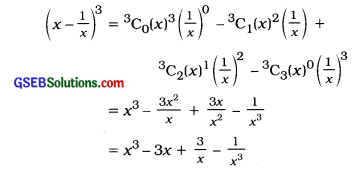Question 15.
Expand : (y + k)5
(y + k)5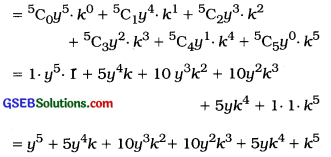Section – D

Question 1.
Using all the first five natural numbers,
(i) how many numbers can be formed?
(ii) how many numbers greater than 30,000 can be formed?
(iii) how many numbers divisible by 5 can be formed?
First five natural numbers are 1 2 3 4 5
(i) Total numbers can be formed = 5P5
= 5!
= 120

(ii) For the numbers greater than 30,000, the digit at the first place can by any one of the digits 3, 4, 5 and remaining 4 places can be arranged from the remaining 4 digits.
.-. Total permutations = 3P1 × 4P4
= 3 × 4!
= 3 × 24
= 72

(iii) For the number divisible by 5, the last digit in the humber should be 5. Remaining 4 places can be arranged from the remaining 4 digits accept digit 5.
∴ Total permutations = 1P1 × 4P4
= 1 × 4!
= 1 × 24 = 24Question 2.
In how many ways can 4 boys and 4 girls be arranged In a row such that no two boys and no two girls appear together?
4 boys and 4 gIrls can be arranged In a row in the following order of arrangements in which two boys and two girls do not appear together.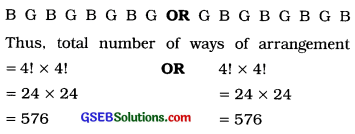Hence, the total number of ways of arranging 4 boys and 4 girls in a row, so that two boys and two girls do not appear together = 576 + 576 = 1152

Question 3.
In how many ways can 3 boys and 2 girls be arranged In a row such that.
(I) both the girls remain together?
(II) boys and girls are alternately arranged?
(III) all the three boys remain together?
3 boys and 2 girls are to be arranged in a row.
(i) Both the girls remain together: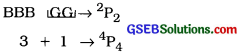∴ Total permutations in which both the girls remain together 4P4 × 2P2
= 4! × 2!
= 24 × 2 = 48

(ii) Boys and girls are alternatively arranged:∴Total permutations in which boys and girls are alternatively arranged
3P3 × 2P2
= 3! × 2!
= 6 × 2
= 12

(iii) All the there boys remain together:∴ Total permutations in which all the three boys remain to gether = 3P3 × 3P3
= 3! × 3!
= 6 × 6
= 36

Question 4.
If all the arrangements formed using all the letters of the word WAKEFUL are arranged in the order of dictionary then what will be the rank of the word WAKEFUL?
7 letters of the word WAKEFUL are W, A, K, E, F, U, L.

These 7 letters can be arranged in 7P7 = 7! = 5040 ways.
Alphabetical order of the letters of the word WAKEFUL is A, E, F, K, L, U, W.

Arrangements with either A, E, F, K, L or U at the first place = 6 [ 1P1 × 6P6]
= 6 [1 × 6!]
= 6 × 720
= 4320

Arrangements with W at first place, A at the second and E at third = 1P1 × 1P1 × 1P1 × 4P4
= 1 × 4!
= 24

Arrangements with W at first place, A at the second and F at third = 1P1 × 1P1 × 1P1 × 4P4
= 1 × 4!
= 24

Now, the word in which W at the first place, A.at the second, K at the third, E at the fourth, F at the fifth.
L at the sixth and U at the seventh is WAKEFLU.

The next word is WAKEFUL.
∴ Dictionary order of the word WAKEFUL is 4320 + 24 + 24 + 1 + 1 = 4370

Question 5.
4 couples (husband-wife) attend a party. In how mpny ways can 2 persons be selected from these 8 persons such that,
(i) two persons selected are husband and wife?
(ii) one is a male and the other is a female?
(iii) one is a male and the other Is a female but they are not husband and wife?
4 couples are there. 2 persons are selected from 8 persons.
(i) Two persons selected are husband and wife:
If selected two persons are husband and wife. then they are one couple of 4 couples.
∴ Total combinations = 4C1 = 4

(iii) One Is a male and the other is a female:
In 8 persons, 4 are males and 4 are females.
One male can be selected in 4C1 ways andone female can be selected In 4C1 ways.
∴ Total combinatíons = 4C1 × 4C1 = 4 × 4 = 16

(iii) One is a male and the other is a female but they are not husband and wife:
Total combinations
[Total combinations in which one is a male and one is female – Total combinations in which they are husband and wife]
= 16 – 4 = 12

Question 6.
There are 4 different books of Statistics and 3 different books of Economics on a table. In how many ways can 2 books be selected such that –
(i) both the books are of the same subject?
(ii) both the books are of different subject?
(iii) no book of Economics is selected?
There are 4 different books of Statistics and 3 different books of Economics on a table.
(i) Both the books are of the same subjects: 2 books of Statistics out of 4 books can be selected in 4C2 ways or 2 books of Economics out of 3 books can be selected in 3C2 ways.
∴ Total combinations = 4C2 + 3C2
= $$\frac{4 \times 3}{2 \times 1}+\frac{3 \times 2}{2 \times 1}$$
= 6 + 3
= 9

(ii) Both the books are of different subject:
one book of Statistics out of 4 books can be selected in 4C1 ways and one book of Economics out of 3 books can be selected in 3C1 ways.
Total combinations = 4C1 × 3C1 = 4 × 3 = 12

(iii) No book of Economics is selected: All two books of statistics out of 4 books can be selected in 4C2 ways and no book of Economics in 3C0 ways.
Total combinations = 4C2 × 3C0
= 6 × 1 = 6

Question 7.
3 dolls, 4 kitchen sets and 3 cars are displayed in a toy shop. In how many ways can 3 toys be selected such that,
(i) all are dolls?
(ii) all are different toys?
(iii) two are dolls and one is a kitchen set?
3 dolls, 4 kitchen sets and 3 cars are displayed in a toy shop. 3 toys are to be selected.
(i) All are dolls : There are 3 dolls.
∴ Total combinations = 3C3 = 1

(ii) All are different toys: One doll out of 3 dolls can be selected in 3C1 ways and one kitchen set out of 4 sets can be selected in 4CX ways and one car out of 3 cars can be selected in 3C1 ways.
∴ Total combinations = 3C1 × 4C1 × 3C1 =3x4x3 = 36

(iii) Two are dolls and one is a kitchen
set: Two dolls out of 3 dolls can be selected in 3C2 ways and one kitchen set out of 4 sets can be selected in 4C1 ways.
∴ Total combinations = 3C2 × 4C1
= $$\frac{3 \times 2}{2 \times 1}$$ × 4
= 3 × 4
= 12Question 8.
A committee of 3 members Is to be formed from 4 chartered accountants and 5 doctors associated with a social organization. In how many ways can the committee be formed such that,
(i) the chartered accountants are in majority?
(ii) the doctors are in majority?
There are 4 CA and 5 doctors. A committee of 3 members Is to be formed.

(i) Chartered accountants are in majority: In a committee of 3 members If chartered accountants are In majority then It can happen In the following two ways:
(a ) 2 CA and 1 doctor OR
(b ) 3 CA and no doctor
2 CA out of 4 and doctor out of 5 can be selected In 4C2 × 5C1 ways. OR
3 CA out of 4 and no doctor out of 5 can be selected in 4C3 × 5C0 ways.

Total combinations = 4C2 × 5C1 + 4C3 × 5C0
= $$\frac{4 \times 3}{2 \times 1}$$ × 5 + 4 × 1
= 30 + 4 = 34

(ii) The doctors are in majority: In a committee of 3 members of the doctors are in majority then it can happen in the following two ways :
(a) 2 doctors and 1 CA OR
(b) 3 doctors and no CA
2 doctors out of 5 and one CA out of 4 can be selected in 5C2 × 4C1 ways OR
3 doctors out of 5 and no CA out of 4 can be selected in 5C3 × 4C0 ways.
Total combinations = 5C2 × 4C1 + 5C3 × 4C0
= $$\frac{5 \times 4}{2 \times 1}$$ × 4 + $$\frac{5 \times 4 \times 3}{3 \times 2 \times 1}$$ × 1
= 40 + 10 = 50

Question 9.
Obtain the value of (√7 + 1)3 – (√7 – 1)3 using binomial expansion method.
(√7 + 1)3 – (√7 – 1)3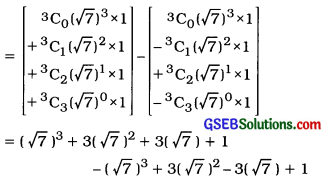= 6 × 7 + 2
= 42 + 2 = 44

Question 10.
Obtain the value of (√3 +1)6 + (√3 – 1)6 using binomial expansion method.